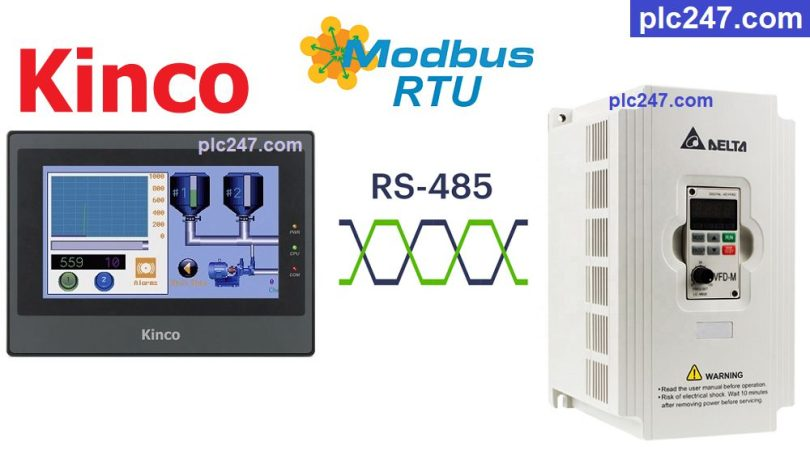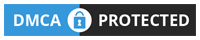# HMI Kinco “Modbus RTU” Delta VFD-M TutorialWritten by

Hello everyone, today plc247.com will write a tutorial for everyone to use HMI Kinco (Model MT4414TE) to connect control and monitor Delta VDF-M inverter in the simplest and easiest way possible.

Both the Kinco HMI and the Delta VFD-M inverter have a built-in RS485 port for Modbus-RTU communication.

#### HMI Kinco & Delta VFD-M “Wiring Diagram”

+ The RS485 port of the Delta VFD-M inverter is the RJ11 port (you can take the desk phone jack as this port)

#### Delta VFD-M “Modbus RTU” Configuration

Below are the communication parameters that need to be set up for Delta VFD-M if we want to control and monitor it using Modbus RTU protocol

• P00: 03 (Frequency Set by RS485)
• P01: 04 ( Control by RS485)
• P89: 01 (Baudrate 9600bps)
• P92: 04 ( Mode 8/1/Even)
• P157: 01 (Modbus-RTU Selection)
##### Note: HMi Kinco Registers Address = “VFD Address (Dec) + 1“

>>> VFD Control Address = 2000 (hex) = 8192 (dec) + 1 = 8193 (dec)

• Set Word 8193 = 18 (dec): Forward Run
• Set Word 8193 = 34 (dec): Reverse Run
• Set Word 8193 = 1 (dec): Stop

>>> Frequency Set Address = 2001 (hex) = 8193 (dec) + 1 = 8194 (dec)

+ Output Frequency Address = 2103 (hex) = 8451 (dec) + 1 = 8452 (dec)

+ Output Current Address = 2104 (hex) = 8452 (dec) + 1 = 8453 (dec)

+ Output Voltage Address = 2106 (hex) = 8454 (dec) + 1 = 8455 (dec)

#### Kinco HMI “Modbus RTU” Parameters Setting

How have you set up the communication parameters for Delta VFD-M inverters, the same settings for HMI Kinco

### HMI Kinco Modbus Example

+ Interface Panel

+ Setting Frequency

+ Run Forward

+ Run Reverse

+ Stop Command

### Detailed Video Tutorial

======

Related Software and Documents